# 9. Regression¶

Chinese proverb

A journey of a thousand miles begins with a single step. – old Chinese proverb

In statistical modeling, regression analysis focuses on investigating the relationship between a dependent variable and one or more independent variables. Wikipedia Regression analysis

In data mining, Regression is a model to represent the relationship between the value of lable ( or target, it is numerical variable) and on one or more features (or predictors they can be numerical and categorical variables).

## 9.1. Linear Regression¶

### 9.1.1. Introduction¶

Given that a data set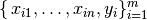which contains n features (variables) and m samples (data points), in simple linear regression model for modeling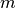data points with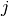independent variables: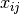, the formula is given by: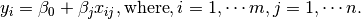In matrix notation, the data set is written as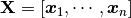with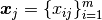,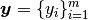(see Fig. Feature matrix and label) and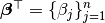. Then the matrix format equation is written as

(1)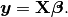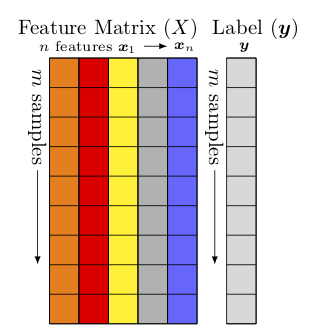Feature matrix and label

### 9.1.2. How to solve it?¶

• For squared or rectangular matrices

• Singular Value Decomposition

• Gram-Schmidt orthogonalization

• QR Decomposition

• For squared matrices

• LU Decomposition

• Cholesky Decomposition

• Regular Splittings

2. Iterative Methods

• Stationary cases iterative method

• Jacobi Method

• Gauss-Seidel Method

• Richardson Method

• Successive Over Relaxation (SOR) Method

• Dynamic cases iterative method

• Chebyshev iterative Method

• Minimal residuals Method

• Minimal correction iterative method

• Steepest Descent Method

### 9.1.3. Ordinary Least Squares¶

In mathematics, (1) is a overdetermined system. The method of ordinary least squares can be used to find an approximate solution to overdetermined systems. For the system overdetermined system (1), the least squares formula is obtained from the problem

(2)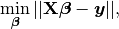the solution of which can be written with the normal equations:

(3)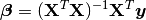where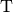indicates a matrix transpose, provided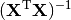exists (that is, provided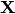has full column rank).

Note

Actually, (3) can be derivated by the following way: multiply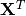on side of (1) and then multiply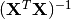on both side of the former result. You may also apply the Extreme Value Theorem to (2) and find the solution (3).

Let’s use the following hypothesis: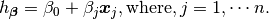Then, solving (2) is equivalent to minimize the following cost fucntion :

### 9.1.5. Cost Function¶

(4)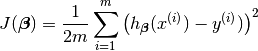Note

The reason why we prefer to solve (4) rather than (2) is because (4) is convex and it has some nice properties, such as it’s uniquely solvable and energy stable for small enough learning rate. the interested reader who has great interest in non-convex cost function (energy) case. is referred to [Feng2016PSD] for more details.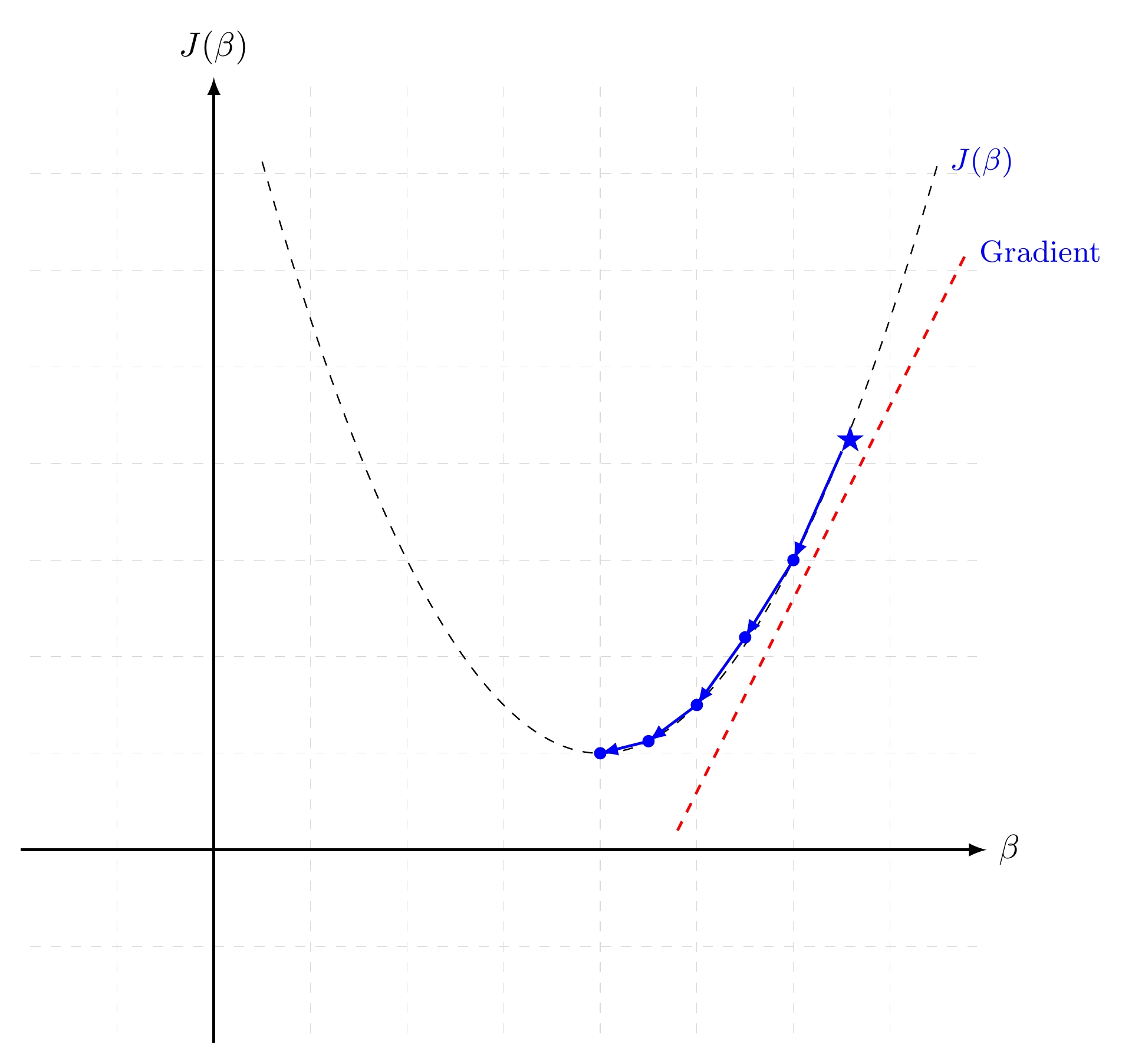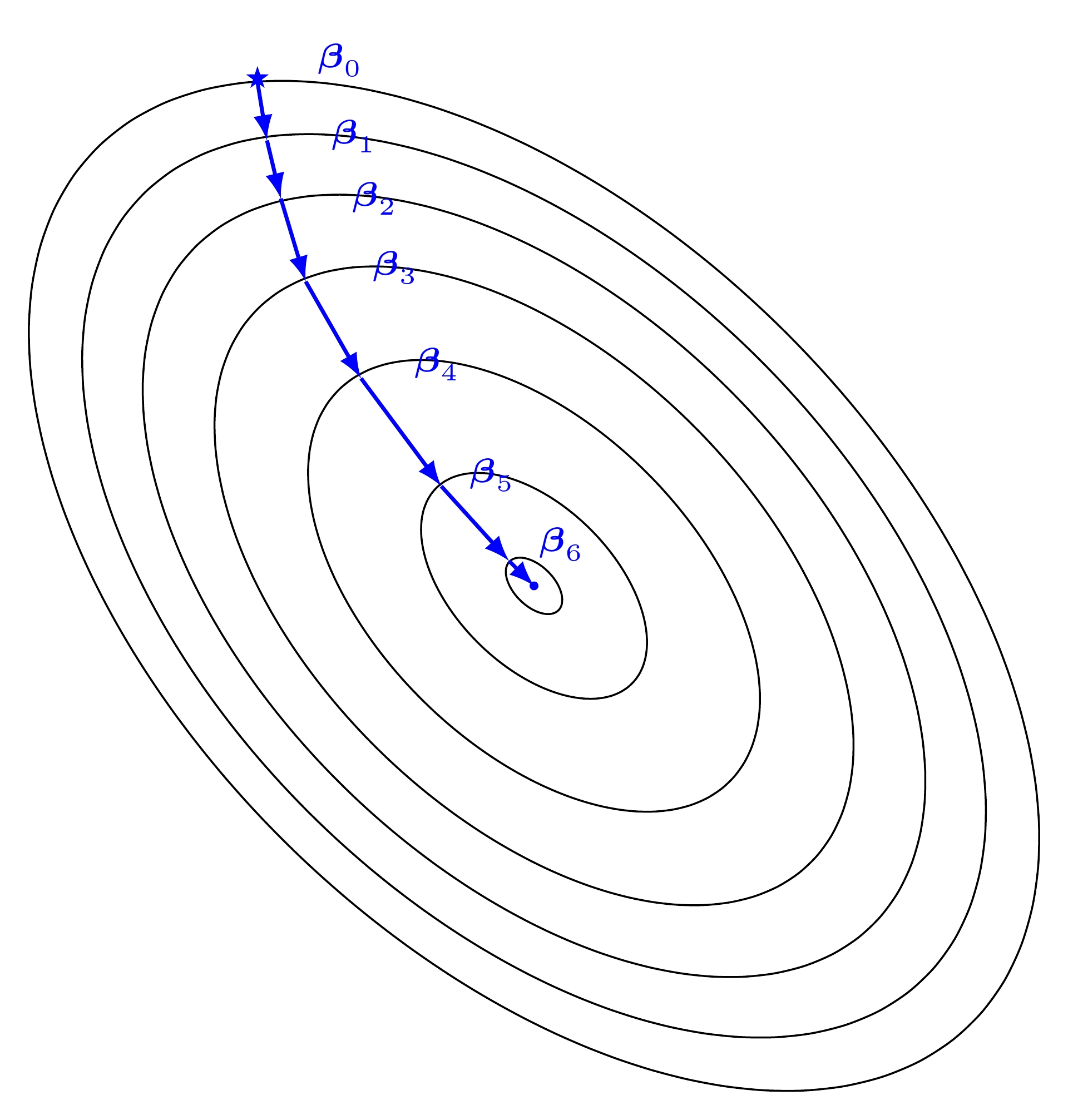Gradient descent is a first-order iterative optimization algorithm for finding the minimum of a function. It searchs with the direction of the steepest desscent which is defined by the negative of the gradient (see Fig. Gradient Descent in 1D and Gradient Descent in 2D for 1D and 2D, respectively) and with learning rate (search step)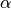.

### 9.1.9. Demo¶

• The Jupyter notebook can be download from Linear Regression which was implemented without using Pipeline.

• The Jupyter notebook can be download from Linear Regression with Pipeline which was implemented with using Pipeline.

• I will only present the code with pipeline style in the following.

• For more details about the parameters, please visit Linear Regression API .

1. Set up spark context and SparkSession

from pyspark.sql import SparkSession

spark = SparkSession \
.builder \
.appName("Python Spark regression example") \
.config("spark.some.config.option", "some-value") \
.getOrCreate()


df = spark.read.format('com.databricks.spark.csv').\
inferschema='true').\


check the data set

df.show(5,True)
df.printSchema()


Then you will get

+-----+-----+---------+-----+
+-----+-----+---------+-----+
|230.1| 37.8|     69.2| 22.1|
| 44.5| 39.3|     45.1| 10.4|
| 17.2| 45.9|     69.3|  9.3|
|151.5| 41.3|     58.5| 18.5|
|180.8| 10.8|     58.4| 12.9|
+-----+-----+---------+-----+
only showing top 5 rows

root
|-- TV: double (nullable = true)
|-- Radio: double (nullable = true)
|-- Newspaper: double (nullable = true)
|-- Sales: double (nullable = true)


You can also get the Statistical results from the data frame (Unfortunately, it only works for numerical).

df.describe().show()


Then you will get

+-------+-----------------+------------------+------------------+------------------+
+-------+-----------------+------------------+------------------+------------------+
|  count|              200|               200|               200|               200|
|   mean|         147.0425|23.264000000000024|30.553999999999995|14.022500000000003|
| stddev|85.85423631490805|14.846809176168728| 21.77862083852283| 5.217456565710477|
|    min|              0.7|               0.0|               0.3|               1.6|
|    max|            296.4|              49.6|             114.0|              27.0|
+-------+-----------------+------------------+------------------+------------------+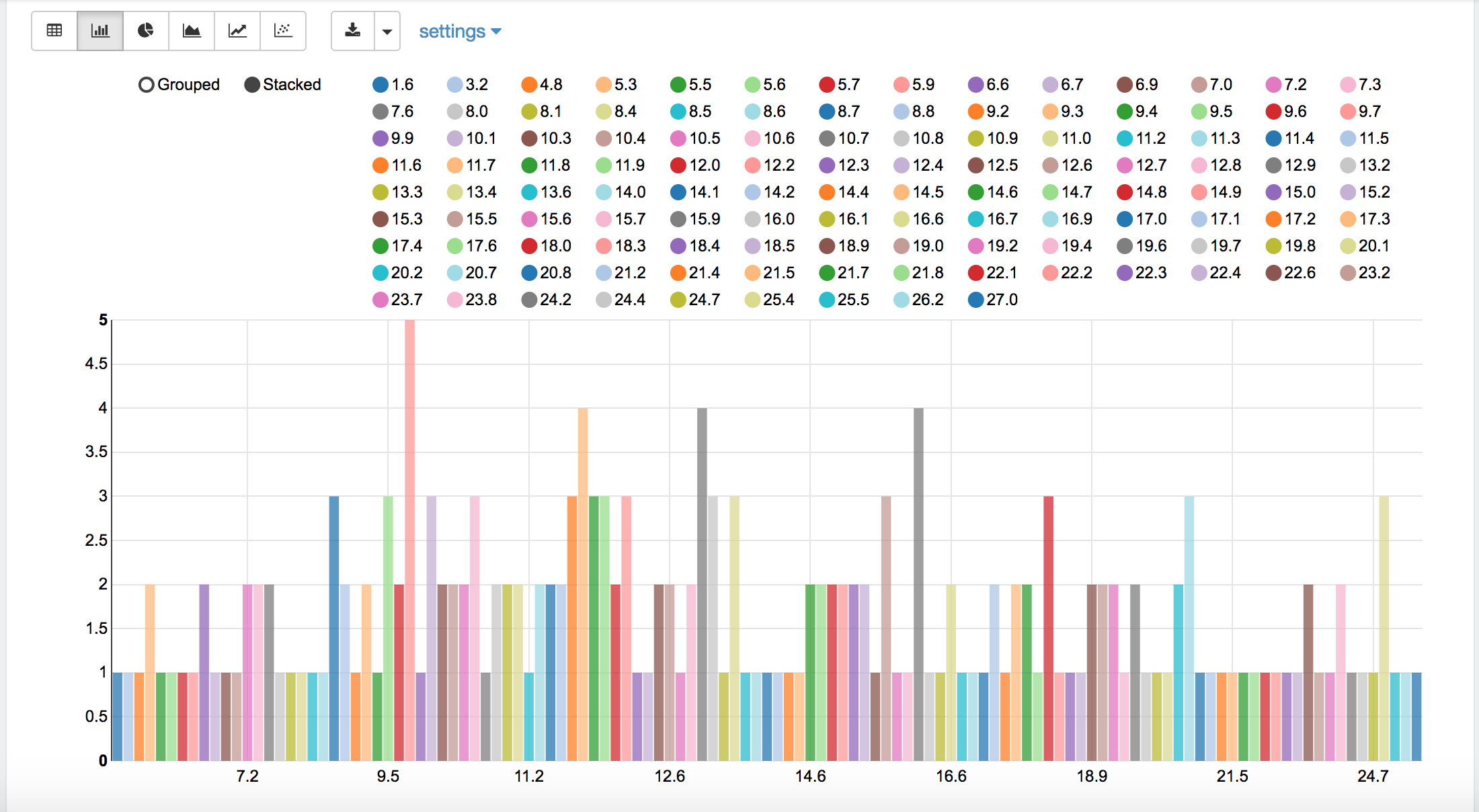Sales distribution

1. Convert the data to dense vector (features and label)

from pyspark.sql import Row
from pyspark.ml.linalg import Vectors

# I provide two ways to build the features and labels

# method 1 (good for small feature):
#def transData(row):
#    return Row(label=row["Sales"],
#               features=Vectors.dense([row["TV"],
#                                       row["Newspaper"]]))

# Method 2 (good for large features):
def transData(data):
return data.rdd.map(lambda r: [Vectors.dense(r[:-1]),r[-1]]).toDF(['features','label'])


Note

You are strongly encouraged to try my get_dummy function for dealing with the categorical data in comple dataset.

Supervised learning version:

def get_dummy(df,indexCol,categoricalCols,continuousCols,labelCol):

from pyspark.ml import Pipeline
from pyspark.ml.feature import StringIndexer, OneHotEncoder, VectorAssembler
from pyspark.sql.functions import col

indexers = [ StringIndexer(inputCol=c, outputCol="{0}_indexed".format(c))
for c in categoricalCols ]

# default setting: dropLast=True
encoders = [ OneHotEncoder(inputCol=indexer.getOutputCol(),
outputCol="{0}_encoded".format(indexer.getOutputCol()))
for indexer in indexers ]

assembler = VectorAssembler(inputCols=[encoder.getOutputCol() for encoder in encoders]
+ continuousCols, outputCol="features")

pipeline = Pipeline(stages=indexers + encoders + [assembler])

model=pipeline.fit(df)
data = model.transform(df)

data = data.withColumn('label',col(labelCol))

if indexCol:
return data.select(indexCol,'features','label')
else:
return data.select('features','label')


Unsupervised learning version:

def get_dummy(df,indexCol,categoricalCols,continuousCols):
'''
Get dummy variables and concat with continuous variables for unsupervised learning.
:param df: the dataframe
:param categoricalCols: the name list of the categorical data
:param continuousCols:  the name list of the numerical data
:return k: feature matrix

:author: Wenqiang Feng
:email:  von198@gmail.com
'''

indexers = [ StringIndexer(inputCol=c, outputCol="{0}_indexed".format(c))
for c in categoricalCols ]

# default setting: dropLast=True
encoders = [ OneHotEncoder(inputCol=indexer.getOutputCol(),
outputCol="{0}_encoded".format(indexer.getOutputCol()))
for indexer in indexers ]

assembler = VectorAssembler(inputCols=[encoder.getOutputCol() for encoder in encoders]
+ continuousCols, outputCol="features")

pipeline = Pipeline(stages=indexers + encoders + [assembler])

model=pipeline.fit(df)
data = model.transform(df)

if indexCol:
return data.select(indexCol,'features')
else:
return data.select('features')


Two in one:

def get_dummy(df,indexCol,categoricalCols,continuousCols,labelCol,dropLast=False):

'''
Get dummy variables and concat with continuous variables for ml modeling.
:param df: the dataframe
:param categoricalCols: the name list of the categorical data
:param continuousCols:  the name list of the numerical data
:param labelCol:  the name of label column
:param dropLast:  the flag of drop last column
:return: feature matrix

:author: Wenqiang Feng
:email:  von198@gmail.com

>>> df = spark.createDataFrame([
(0, "a"),
(1, "b"),
(2, "c"),
(3, "a"),
(4, "a"),
(5, "c")
], ["id", "category"])

>>> indexCol = 'id'
>>> categoricalCols = ['category']
>>> continuousCols = []
>>> labelCol = []

>>> mat = get_dummy(df,indexCol,categoricalCols,continuousCols,labelCol)
>>> mat.show()

>>>
+---+-------------+
| id|     features|
+---+-------------+
|  0|[1.0,0.0,0.0]|
|  1|[0.0,0.0,1.0]|
|  2|[0.0,1.0,0.0]|
|  3|[1.0,0.0,0.0]|
|  4|[1.0,0.0,0.0]|
|  5|[0.0,1.0,0.0]|
+---+-------------+
'''

from pyspark.ml import Pipeline
from pyspark.ml.feature import StringIndexer, OneHotEncoder, VectorAssembler
from pyspark.sql.functions import col

indexers = [ StringIndexer(inputCol=c, outputCol="{0}_indexed".format(c))
for c in categoricalCols ]

# default setting: dropLast=True
encoders = [ OneHotEncoder(inputCol=indexer.getOutputCol(),
outputCol="{0}_encoded".format(indexer.getOutputCol()),dropLast=dropLast)
for indexer in indexers ]

assembler = VectorAssembler(inputCols=[encoder.getOutputCol() for encoder in encoders]
+ continuousCols, outputCol="features")

pipeline = Pipeline(stages=indexers + encoders + [assembler])

model=pipeline.fit(df)
data = model.transform(df)

if indexCol and labelCol:
# for supervised learning
data = data.withColumn('label',col(labelCol))
return data.select(indexCol,'features','label')
elif not indexCol and labelCol:
# for supervised learning
data = data.withColumn('label',col(labelCol))
return data.select('features','label')
elif indexCol and not labelCol:
# for unsupervised learning
return data.select(indexCol,'features')
elif not indexCol and not labelCol:
# for unsupervised learning
return data.select('features')

1. Transform the dataset to DataFrame

transformed= transData(df)
transformed.show(5)

+-----------------+-----+
|         features|label|
+-----------------+-----+
|[230.1,37.8,69.2]| 22.1|
| [44.5,39.3,45.1]| 10.4|
| [17.2,45.9,69.3]|  9.3|
|[151.5,41.3,58.5]| 18.5|
|[180.8,10.8,58.4]| 12.9|
+-----------------+-----+
only showing top 5 rows


Note

You will find out that all of the supervised machine learning algorithms in Spark are based on the features and label (unsupervised machine learning algorithms in Spark are based on the features). That is to say, you can play with all of the machine learning algorithms in Spark when you get ready the features and label in pipeline architecture.

1. Deal With Categorical Variables

from pyspark.ml import Pipeline
from pyspark.ml.regression import LinearRegression
from pyspark.ml.feature import VectorIndexer
from pyspark.ml.evaluation import RegressionEvaluator

# Automatically identify categorical features, and index them.
# We specify maxCategories so features with > 4 distinct values are treated as continuous.

featureIndexer = VectorIndexer(inputCol="features", \
outputCol="indexedFeatures",\
maxCategories=4).fit(transformed)

data = featureIndexer.transform(transformed)


Now you check your dataset with

data.show(5,True)


you will get

+-----------------+-----+-----------------+
|         features|label|  indexedFeatures|
+-----------------+-----+-----------------+
|[230.1,37.8,69.2]| 22.1|[230.1,37.8,69.2]|
| [44.5,39.3,45.1]| 10.4| [44.5,39.3,45.1]|
| [17.2,45.9,69.3]|  9.3| [17.2,45.9,69.3]|
|[151.5,41.3,58.5]| 18.5|[151.5,41.3,58.5]|
|[180.8,10.8,58.4]| 12.9|[180.8,10.8,58.4]|
+-----------------+-----+-----------------+
only showing top 5 rows

1. Split the data into training and test sets (40% held out for testing)

# Split the data into training and test sets (40% held out for testing)
(trainingData, testData) = transformed.randomSplit([0.6, 0.4])


You can check your train and test data as follows (In my opinion, it is always to good to keep tracking your data during prototype pahse):

trainingData.show(5)
testData.show(5)


Then you will get

+---------------+-----+---------------+
|       features|label|indexedFeatures|
+---------------+-----+---------------+
| [4.1,11.6,5.7]|  3.2| [4.1,11.6,5.7]|
| [5.4,29.9,9.4]|  5.3| [5.4,29.9,9.4]|
|[7.3,28.1,41.4]|  5.5|[7.3,28.1,41.4]|
|[7.8,38.9,50.6]|  6.6|[7.8,38.9,50.6]|
|  [8.6,2.1,1.0]|  4.8|  [8.6,2.1,1.0]|
+---------------+-----+---------------+
only showing top 5 rows

+----------------+-----+----------------+
|        features|label| indexedFeatures|
+----------------+-----+----------------+
|  [0.7,39.6,8.7]|  1.6|  [0.7,39.6,8.7]|
|  [8.4,27.2,2.1]|  5.7|  [8.4,27.2,2.1]|
|[11.7,36.9,45.2]|  7.3|[11.7,36.9,45.2]|
|[13.2,15.9,49.6]|  5.6|[13.2,15.9,49.6]|
|[16.9,43.7,89.4]|  8.7|[16.9,43.7,89.4]|
+----------------+-----+----------------+
only showing top 5 rows

1. Fit Ordinary Least Square Regression Model

For more details about the parameters, please visit Linear Regression API .

# Import LinearRegression class
from pyspark.ml.regression import LinearRegression

# Define LinearRegression algorithm
lr = LinearRegression()

1. Pipeline Architecture

# Chain indexer and tree in a Pipeline
pipeline = Pipeline(stages=[featureIndexer, lr])

model = pipeline.fit(trainingData)

1. Summary of the Model

Spark has a poor summary function for data and model. I wrote a summary function which has similar format as R output for the linear regression in PySpark.

def modelsummary(model):
import numpy as np
print ("Note: the last rows are the information for Intercept")
print ("##","-------------------------------------------------")
print ("##","  Estimate   |   Std.Error | t Values  |  P-value")
coef = np.append(list(model.coefficients),model.intercept)
Summary=model.summary

for i in range(len(Summary.pValues)):
print ("##",'{:10.6f}'.format(coef[i]),\
'{:10.6f}'.format(Summary.coefficientStandardErrors[i]),\
'{:8.3f}'.format(Summary.tValues[i]),\
'{:10.6f}'.format(Summary.pValues[i]))

print ("##",'---')
print ("##","Mean squared error: % .6f" \
% Summary.meanSquaredError, ", RMSE: % .6f" \
% Summary.rootMeanSquaredError )
print ("##","Multiple R-squared: %f" % Summary.r2, ", \
Total iterations: %i"% Summary.totalIterations)

modelsummary(model.stages[-1])


You will get the following summary results:

Note: the last rows are the information for Intercept
('##', '-------------------------------------------------')
('##', '  Estimate   |   Std.Error | t Values  |  P-value')
('##', '  0.044186', '  0.001663', '  26.573', '  0.000000')
('##', '  0.206311', '  0.010846', '  19.022', '  0.000000')
('##', '  0.001963', '  0.007467', '   0.263', '  0.793113')
('##', '  2.596154', '  0.379550', '   6.840', '  0.000000')
('##', '---')
('##', 'Mean squared error:  2.588230', ', RMSE:  1.608798')
('##', 'Multiple R-squared: 0.911869', ',             Total iterations: 1')

1. Make predictions

# Make predictions.
predictions = model.transform(testData)

# Select example rows to display.
predictions.select("features","label","prediction").show(5)

+----------------+-----+------------------+
|        features|label|        prediction|
+----------------+-----+------------------+
|  [0.7,39.6,8.7]|  1.6| 10.81405928637388|
|  [8.4,27.2,2.1]|  5.7| 8.583086404079918|
|[11.7,36.9,45.2]|  7.3|10.814712818232422|
|[13.2,15.9,49.6]|  5.6| 6.557106943899219|
|[16.9,43.7,89.4]|  8.7|12.534151375058645|
+----------------+-----+------------------+
only showing top 5 rows

1. Evaluation

from pyspark.ml.evaluation import RegressionEvaluator
# Select (prediction, true label) and compute test error
evaluator = RegressionEvaluator(labelCol="label",
predictionCol="prediction",
metricName="rmse")

rmse = evaluator.evaluate(predictions)
print("Root Mean Squared Error (RMSE) on test data = %g" % rmse)


The final Root Mean Squared Error (RMSE) is as follows:

Root Mean Squared Error (RMSE) on test data = 1.63114


You can also check the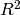value for the test data:

y_true = predictions.select("label").toPandas()
y_pred = predictions.select("prediction").toPandas()

import sklearn.metrics
r2_score = sklearn.metrics.r2_score(y_true, y_pred)
print('r2_score: {0}'.format(r2_score))


Then you will get

r2_score: 0.854486655585


Warning

You should know most softwares are using different formula to calculate thevalue when no intercept is included in the model. You can get more information from the disscussion at StackExchange.

## 9.2. Generalized linear regression¶

### 9.2.3. Demo¶

1. Set up spark context and SparkSession

from pyspark.sql import SparkSession

spark = SparkSession \
.builder \
.appName("Python Spark regression example") \
.config("spark.some.config.option", "some-value") \
.getOrCreate()


df = spark.read.format('com.databricks.spark.csv').\
inferschema='true').\


check the data set

df.show(5,True)
df.printSchema()


Then you will get

+-----+-----+---------+-----+
+-----+-----+---------+-----+
|230.1| 37.8|     69.2| 22.1|
| 44.5| 39.3|     45.1| 10.4|
| 17.2| 45.9|     69.3|  9.3|
|151.5| 41.3|     58.5| 18.5|
|180.8| 10.8|     58.4| 12.9|
+-----+-----+---------+-----+
only showing top 5 rows

root
|-- TV: double (nullable = true)
|-- Radio: double (nullable = true)
|-- Newspaper: double (nullable = true)
|-- Sales: double (nullable = true)


You can also get the Statistical resutls from the data frame (Unfortunately, it only works for numerical).

df.describe().show()


Then you will get

+-------+-----------------+------------------+------------------+------------------+
+-------+-----------------+------------------+------------------+------------------+
|  count|              200|               200|               200|               200|
|   mean|         147.0425|23.264000000000024|30.553999999999995|14.022500000000003|
| stddev|85.85423631490805|14.846809176168728| 21.77862083852283| 5.217456565710477|
|    min|              0.7|               0.0|               0.3|               1.6|
|    max|            296.4|              49.6|             114.0|              27.0|
+-------+-----------------+------------------+------------------+------------------+

1. Convert the data to dense vector (features and label)

Note

You are strongly encouraged to try my get_dummy function for dealing with the categorical data in comple dataset.

Supervised learning version:

def get_dummy(df,indexCol,categoricalCols,continuousCols,labelCol):

from pyspark.ml import Pipeline
from pyspark.ml.feature import StringIndexer, OneHotEncoder, VectorAssembler
from pyspark.sql.functions import col

indexers = [ StringIndexer(inputCol=c, outputCol="{0}_indexed".format(c))
for c in categoricalCols ]

# default setting: dropLast=True
encoders = [ OneHotEncoder(inputCol=indexer.getOutputCol(),
outputCol="{0}_encoded".format(indexer.getOutputCol()))
for indexer in indexers ]

assembler = VectorAssembler(inputCols=[encoder.getOutputCol() for encoder in encoders]
+ continuousCols, outputCol="features")

pipeline = Pipeline(stages=indexers + encoders + [assembler])

model=pipeline.fit(df)
data = model.transform(df)

data = data.withColumn('label',col(labelCol))

if indexCol:
return data.select(indexCol,'features','label')
else:
return data.select('features','label')


Unsupervised learning version:

def get_dummy(df,indexCol,categoricalCols,continuousCols):
'''
Get dummy variables and concat with continuous variables for unsupervised learning.
:param df: the dataframe
:param categoricalCols: the name list of the categorical data
:param continuousCols:  the name list of the numerical data
:return k: feature matrix

:author: Wenqiang Feng
:email:  von198@gmail.com
'''

indexers = [ StringIndexer(inputCol=c, outputCol="{0}_indexed".format(c))
for c in categoricalCols ]

# default setting: dropLast=True
encoders = [ OneHotEncoder(inputCol=indexer.getOutputCol(),
outputCol="{0}_encoded".format(indexer.getOutputCol()))
for indexer in indexers ]

assembler = VectorAssembler(inputCols=[encoder.getOutputCol() for encoder in encoders]
+ continuousCols, outputCol="features")

pipeline = Pipeline(stages=indexers + encoders + [assembler])

model=pipeline.fit(df)
data = model.transform(df)

if indexCol:
return data.select(indexCol,'features')
else:
return data.select('features')


Two in one:

def get_dummy(df,indexCol,categoricalCols,continuousCols,labelCol,dropLast=False):

'''
Get dummy variables and concat with continuous variables for ml modeling.
:param df: the dataframe
:param categoricalCols: the name list of the categorical data
:param continuousCols:  the name list of the numerical data
:param labelCol:  the name of label column
:param dropLast:  the flag of drop last column
:return: feature matrix

:author: Wenqiang Feng
:email:  von198@gmail.com

>>> df = spark.createDataFrame([
(0, "a"),
(1, "b"),
(2, "c"),
(3, "a"),
(4, "a"),
(5, "c")
], ["id", "category"])

>>> indexCol = 'id'
>>> categoricalCols = ['category']
>>> continuousCols = []
>>> labelCol = []

>>> mat = get_dummy(df,indexCol,categoricalCols,continuousCols,labelCol)
>>> mat.show()

>>>
+---+-------------+
| id|     features|
+---+-------------+
|  0|[1.0,0.0,0.0]|
|  1|[0.0,0.0,1.0]|
|  2|[0.0,1.0,0.0]|
|  3|[1.0,0.0,0.0]|
|  4|[1.0,0.0,0.0]|
|  5|[0.0,1.0,0.0]|
+---+-------------+
'''

from pyspark.ml import Pipeline
from pyspark.ml.feature import StringIndexer, OneHotEncoder, VectorAssembler
from pyspark.sql.functions import col

indexers = [ StringIndexer(inputCol=c, outputCol="{0}_indexed".format(c))
for c in categoricalCols ]

# default setting: dropLast=True
encoders = [ OneHotEncoder(inputCol=indexer.getOutputCol(),
outputCol="{0}_encoded".format(indexer.getOutputCol()),dropLast=dropLast)
for indexer in indexers ]

assembler = VectorAssembler(inputCols=[encoder.getOutputCol() for encoder in encoders]
+ continuousCols, outputCol="features")

pipeline = Pipeline(stages=indexers + encoders + [assembler])

model=pipeline.fit(df)
data = model.transform(df)

if indexCol and labelCol:
# for supervised learning
data = data.withColumn('label',col(labelCol))
return data.select(indexCol,'features','label')
elif not indexCol and labelCol:
# for supervised learning
data = data.withColumn('label',col(labelCol))
return data.select('features','label')
elif indexCol and not labelCol:
# for unsupervised learning
return data.select(indexCol,'features')
elif not indexCol and not labelCol:
# for unsupervised learning
return data.select('features')

from pyspark.sql import Row
from pyspark.ml.linalg import Vectors

# I provide two ways to build the features and labels

# method 1 (good for small feature):
#def transData(row):
#    return Row(label=row["Sales"],
#               features=Vectors.dense([row["TV"],
#                                       row["Newspaper"]]))

# Method 2 (good for large features):
def transData(data):
return data.rdd.map(lambda r: [Vectors.dense(r[:-1]),r[-1]]).toDF(['features','label'])

transformed= transData(df)
transformed.show(5)

+-----------------+-----+
|         features|label|
+-----------------+-----+
|[230.1,37.8,69.2]| 22.1|
| [44.5,39.3,45.1]| 10.4|
| [17.2,45.9,69.3]|  9.3|
|[151.5,41.3,58.5]| 18.5|
|[180.8,10.8,58.4]| 12.9|
+-----------------+-----+
only showing top 5 rows


Note

You will find out that all of the machine learning algorithms in Spark are based on the features and label. That is to say, you can play with all of the machine learning algorithms in Spark when you get ready the features and label.

1. Convert the data to dense vector

# convert the data to dense vector
def transData(data):
return data.rdd.map(lambda r: [r[-1], Vectors.dense(r[:-1])]).\
toDF(['label','features'])

from pyspark.sql import Row
from pyspark.ml.linalg import Vectors

data= transData(df)
data.show()

1. Deal with the Categorical variables

from pyspark.ml import Pipeline
from pyspark.ml.regression import LinearRegression
from pyspark.ml.feature import VectorIndexer
from pyspark.ml.evaluation import RegressionEvaluator

# Automatically identify categorical features, and index them.
# We specify maxCategories so features with > 4
# distinct values are treated as continuous.

featureIndexer = VectorIndexer(inputCol="features", \
outputCol="indexedFeatures",\
maxCategories=4).fit(transformed)

data = featureIndexer.transform(transformed)


When you check you data at this point, you will get

+-----------------+-----+-----------------+
|         features|label|  indexedFeatures|
+-----------------+-----+-----------------+
|[230.1,37.8,69.2]| 22.1|[230.1,37.8,69.2]|
| [44.5,39.3,45.1]| 10.4| [44.5,39.3,45.1]|
| [17.2,45.9,69.3]|  9.3| [17.2,45.9,69.3]|
|[151.5,41.3,58.5]| 18.5|[151.5,41.3,58.5]|
|[180.8,10.8,58.4]| 12.9|[180.8,10.8,58.4]|
+-----------------+-----+-----------------+
only showing top 5 rows

1. Split the data into training and test sets (40% held out for testing)

# Split the data into training and test sets (40% held out for testing)
(trainingData, testData) = transformed.randomSplit([0.6, 0.4])


You can check your train and test data as follows (In my opinion, it is always to good to keep tracking your data during prototype phase):

trainingData.show(5)
testData.show(5)


Then you will get

+----------------+-----+----------------+
|        features|label| indexedFeatures|
+----------------+-----+----------------+
|  [5.4,29.9,9.4]|  5.3|  [5.4,29.9,9.4]|
| [7.8,38.9,50.6]|  6.6| [7.8,38.9,50.6]|
|  [8.4,27.2,2.1]|  5.7|  [8.4,27.2,2.1]|
| [8.7,48.9,75.0]|  7.2| [8.7,48.9,75.0]|
|[11.7,36.9,45.2]|  7.3|[11.7,36.9,45.2]|
+----------------+-----+----------------+
only showing top 5 rows

+---------------+-----+---------------+
|       features|label|indexedFeatures|
+---------------+-----+---------------+
| [0.7,39.6,8.7]|  1.6| [0.7,39.6,8.7]|
| [4.1,11.6,5.7]|  3.2| [4.1,11.6,5.7]|
|[7.3,28.1,41.4]|  5.5|[7.3,28.1,41.4]|
|  [8.6,2.1,1.0]|  4.8|  [8.6,2.1,1.0]|
|[17.2,4.1,31.6]|  5.9|[17.2,4.1,31.6]|
+---------------+-----+---------------+
only showing top 5 rows

1. Fit Generalized Linear Regression Model

# Import LinearRegression class
from pyspark.ml.regression import GeneralizedLinearRegression

# Define LinearRegression algorithm
maxIter=10, regParam=0.3)

1. Pipeline Architecture

# Chain indexer and tree in a Pipeline
pipeline = Pipeline(stages=[featureIndexer, glr])

model = pipeline.fit(trainingData)

1. Summary of the Model

Spark has a poor summary function for data and model. I wrote a summary function which has similar format as R output for the linear regression in PySpark.

def modelsummary(model):
import numpy as np
print ("Note: the last rows are the information for Intercept")
print ("##","-------------------------------------------------")
print ("##","  Estimate   |   Std.Error | t Values  |  P-value")
coef = np.append(list(model.coefficients),model.intercept)
Summary=model.summary

for i in range(len(Summary.pValues)):
print ("##",'{:10.6f}'.format(coef[i]),\
'{:10.6f}'.format(Summary.coefficientStandardErrors[i]),\
'{:8.3f}'.format(Summary.tValues[i]),\
'{:10.6f}'.format(Summary.pValues[i]))

print ("##",'---')
#     print ("##","Mean squared error: % .6f" \
#            % Summary.meanSquaredError, ", RMSE: % .6f" \
#            % Summary.rootMeanSquaredError )
#     print ("##","Multiple R-squared: %f" % Summary.r2, ", \
#             Total iterations: %i"% Summary.totalIterations)

modelsummary(model.stages[-1])


You will get the following summary results:

Note: the last rows are the information for Intercept
('##', '-------------------------------------------------')
('##', '  Estimate   |   Std.Error | t Values  |  P-value')
('##', '  0.042857', '  0.001668', '  25.692', '  0.000000')
('##', '  0.199922', '  0.009881', '  20.232', '  0.000000')
('##', ' -0.001957', '  0.006917', '  -0.283', '  0.777757')
('##', '  3.007515', '  0.406389', '   7.401', '  0.000000')
('##', '---')

1. Make predictions

# Make predictions.
predictions = model.transform(testData)

# Select example rows to display.
predictions.select("features","label","predictedLabel").show(5)

+---------------+-----+------------------+
|       features|label|        prediction|
+---------------+-----+------------------+
| [0.7,39.6,8.7]|  1.6|10.937383732327625|
| [4.1,11.6,5.7]|  3.2| 5.491166258750164|
|[7.3,28.1,41.4]|  5.5|   8.8571603947873|
|  [8.6,2.1,1.0]|  4.8| 3.793966281660073|
|[17.2,4.1,31.6]|  5.9| 4.502507124763654|
+---------------+-----+------------------+
only showing top 5 rows

1. Evaluation

from pyspark.ml.evaluation import RegressionEvaluator
from pyspark.ml.evaluation import RegressionEvaluator
# Select (prediction, true label) and compute test error
evaluator = RegressionEvaluator(labelCol="label",
predictionCol="prediction",
metricName="rmse")

rmse = evaluator.evaluate(predictions)
print("Root Mean Squared Error (RMSE) on test data = %g" % rmse)


The final Root Mean Squared Error (RMSE) is as follows:

Root Mean Squared Error (RMSE) on test data = 1.89857

y_true = predictions.select("label").toPandas()
y_pred = predictions.select("prediction").toPandas()

import sklearn.metrics
r2_score = sklearn.metrics.r2_score(y_true, y_pred)
print('r2_score: {0}'.format(r2_score))


Then you will get thevalue:

r2_score: 0.87707391843


## 9.3. Decision tree Regression¶

### 9.3.3. Demo¶

1. Set up spark context and SparkSession

from pyspark.sql import SparkSession

spark = SparkSession \
.builder \
.appName("Python Spark regression example") \
.config("spark.some.config.option", "some-value") \
.getOrCreate()


df = spark.read.format('com.databricks.spark.csv').\
inferschema='true').\


check the data set

df.show(5,True)
df.printSchema()


Then you will get

+-----+-----+---------+-----+
+-----+-----+---------+-----+
|230.1| 37.8|     69.2| 22.1|
| 44.5| 39.3|     45.1| 10.4|
| 17.2| 45.9|     69.3|  9.3|
|151.5| 41.3|     58.5| 18.5|
|180.8| 10.8|     58.4| 12.9|
+-----+-----+---------+-----+
only showing top 5 rows

root
|-- TV: double (nullable = true)
|-- Radio: double (nullable = true)
|-- Newspaper: double (nullable = true)
|-- Sales: double (nullable = true)


You can also get the Statistical resutls from the data frame (Unfortunately, it only works for numerical).

df.describe().show()


Then you will get

+-------+-----------------+------------------+------------------+------------------+
+-------+-----------------+------------------+------------------+------------------+
|  count|              200|               200|               200|               200|
|   mean|         147.0425|23.264000000000024|30.553999999999995|14.022500000000003|
| stddev|85.85423631490805|14.846809176168728| 21.77862083852283| 5.217456565710477|
|    min|              0.7|               0.0|               0.3|               1.6|
|    max|            296.4|              49.6|             114.0|              27.0|
+-------+-----------------+------------------+------------------+------------------+

1. Convert the data to dense vector (features and label)

Note

You are strongly encouraged to try my get_dummy function for dealing with the categorical data in comple dataset.

Supervised learning version:

def get_dummy(df,indexCol,categoricalCols,continuousCols,labelCol):

from pyspark.ml import Pipeline
from pyspark.ml.feature import StringIndexer, OneHotEncoder, VectorAssembler
from pyspark.sql.functions import col

indexers = [ StringIndexer(inputCol=c, outputCol="{0}_indexed".format(c))
for c in categoricalCols ]

# default setting: dropLast=True
encoders = [ OneHotEncoder(inputCol=indexer.getOutputCol(),
outputCol="{0}_encoded".format(indexer.getOutputCol()))
for indexer in indexers ]

assembler = VectorAssembler(inputCols=[encoder.getOutputCol() for encoder in encoders]
+ continuousCols, outputCol="features")

pipeline = Pipeline(stages=indexers + encoders + [assembler])

model=pipeline.fit(df)
data = model.transform(df)

data = data.withColumn('label',col(labelCol))

return data.select(indexCol,'features','label')


Unsupervised learning version:

def get_dummy(df,indexCol,categoricalCols,continuousCols):
'''
Get dummy variables and concat with continuous variables for unsupervised learning.
:param df: the dataframe
:param categoricalCols: the name list of the categorical data
:param continuousCols:  the name list of the numerical data
:return k: feature matrix

:author: Wenqiang Feng
:email:  von198@gmail.com
'''

indexers = [ StringIndexer(inputCol=c, outputCol="{0}_indexed".format(c))
for c in categoricalCols ]

# default setting: dropLast=True
encoders = [ OneHotEncoder(inputCol=indexer.getOutputCol(),
outputCol="{0}_encoded".format(indexer.getOutputCol()))
for indexer in indexers ]

assembler = VectorAssembler(inputCols=[encoder.getOutputCol() for encoder in encoders]
+ continuousCols, outputCol="features")

pipeline = Pipeline(stages=indexers + encoders + [assembler])

model=pipeline.fit(df)
data = model.transform(df)

return data.select(indexCol,'features')


Two in one:

def get_dummy(df,indexCol,categoricalCols,continuousCols,labelCol,dropLast=False):

'''
Get dummy variables and concat with continuous variables for ml modeling.
:param df: the dataframe
:param categoricalCols: the name list of the categorical data
:param continuousCols:  the name list of the numerical data
:param labelCol:  the name of label column
:param dropLast:  the flag of drop last column
:return: feature matrix

:author: Wenqiang Feng
:email:  von198@gmail.com

>>> df = spark.createDataFrame([
(0, "a"),
(1, "b"),
(2, "c"),
(3, "a"),
(4, "a"),
(5, "c")
], ["id", "category"])

>>> indexCol = 'id'
>>> categoricalCols = ['category']
>>> continuousCols = []
>>> labelCol = []

>>> mat = get_dummy(df,indexCol,categoricalCols,continuousCols,labelCol)
>>> mat.show()

>>>
+---+-------------+
| id|     features|
+---+-------------+
|  0|[1.0,0.0,0.0]|
|  1|[0.0,0.0,1.0]|
|  2|[0.0,1.0,0.0]|
|  3|[1.0,0.0,0.0]|
|  4|[1.0,0.0,0.0]|
|  5|[0.0,1.0,0.0]|
+---+-------------+
'''

from pyspark.ml import Pipeline
from pyspark.ml.feature import StringIndexer, OneHotEncoder, VectorAssembler
from pyspark.sql.functions import col

indexers = [ StringIndexer(inputCol=c, outputCol="{0}_indexed".format(c))
for c in categoricalCols ]

# default setting: dropLast=True
encoders = [ OneHotEncoder(inputCol=indexer.getOutputCol(),
outputCol="{0}_encoded".format(indexer.getOutputCol()),dropLast=dropLast)
for indexer in indexers ]

assembler = VectorAssembler(inputCols=[encoder.getOutputCol() for encoder in encoders]
+ continuousCols, outputCol="features")

pipeline = Pipeline(stages=indexers + encoders + [assembler])

model=pipeline.fit(df)
data = model.transform(df)

if indexCol and labelCol:
# for supervised learning
data = data.withColumn('label',col(labelCol))
return data.select(indexCol,'features','label')
elif not indexCol and labelCol:
# for supervised learning
data = data.withColumn('label',col(labelCol))
return data.select('features','label')
elif indexCol and not labelCol:
# for unsupervised learning
return data.select(indexCol,'features')
elif not indexCol and not labelCol:
# for unsupervised learning
return data.select('features')

from pyspark.sql import Row
from pyspark.ml.linalg import Vectors

# I provide two ways to build the features and labels

# method 1 (good for small feature):
#def transData(row):
#    return Row(label=row["Sales"],
#               features=Vectors.dense([row["TV"],
#                                       row["Newspaper"]]))

# Method 2 (good for large features):
def transData(data):
return data.rdd.map(lambda r: [Vectors.dense(r[:-1]),r[-1]]).toDF(['features','label'])

transformed= transData(df)
transformed.show(5)

+-----------------+-----+
|         features|label|
+-----------------+-----+
|[230.1,37.8,69.2]| 22.1|
| [44.5,39.3,45.1]| 10.4|
| [17.2,45.9,69.3]|  9.3|
|[151.5,41.3,58.5]| 18.5|
|[180.8,10.8,58.4]| 12.9|
+-----------------+-----+
only showing top 5 rows


Note

You will find out that all of the machine learning algorithms in Spark are based on the features and label. That is to say, you can play with all of the machine learning algorithms in Spark when you get ready the features and label.

1. Convert the data to dense vector

# convert the data to dense vector
def transData(data):
return data.rdd.map(lambda r: [r[-1], Vectors.dense(r[:-1])]).\
toDF(['label','features'])

transformed = transData(df)
transformed.show(5)

1. Deal with the Categorical variables

from pyspark.ml import Pipeline
from pyspark.ml.regression import LinearRegression
from pyspark.ml.feature import VectorIndexer
from pyspark.ml.evaluation import RegressionEvaluator

# Automatically identify categorical features, and index them.
# We specify maxCategories so features with > 4
# distinct values are treated as continuous.

featureIndexer = VectorIndexer(inputCol="features", \
outputCol="indexedFeatures",\
maxCategories=4).fit(transformed)

data = featureIndexer.transform(transformed)


When you check you data at this point, you will get

+-----------------+-----+-----------------+
|         features|label|  indexedFeatures|
+-----------------+-----+-----------------+
|[230.1,37.8,69.2]| 22.1|[230.1,37.8,69.2]|
| [44.5,39.3,45.1]| 10.4| [44.5,39.3,45.1]|
| [17.2,45.9,69.3]|  9.3| [17.2,45.9,69.3]|
|[151.5,41.3,58.5]| 18.5|[151.5,41.3,58.5]|
|[180.8,10.8,58.4]| 12.9|[180.8,10.8,58.4]|
+-----------------+-----+-----------------+
only showing top 5 rows

1. Split the data into training and test sets (40% held out for testing)

# Split the data into training and test sets (40% held out for testing)
(trainingData, testData) = transformed.randomSplit([0.6, 0.4])


You can check your train and test data as follows (In my opinion, it is always to good to keep tracking your data during prototype pahse):

trainingData.show(5)
testData.show(5)


Then you will get

+---------------+-----+---------------+
|       features|label|indexedFeatures|
+---------------+-----+---------------+
| [4.1,11.6,5.7]|  3.2| [4.1,11.6,5.7]|
|[7.3,28.1,41.4]|  5.5|[7.3,28.1,41.4]|
| [8.4,27.2,2.1]|  5.7| [8.4,27.2,2.1]|
|  [8.6,2.1,1.0]|  4.8|  [8.6,2.1,1.0]|
|[8.7,48.9,75.0]|  7.2|[8.7,48.9,75.0]|
+---------------+-----+---------------+
only showing top 5 rows

+----------------+-----+----------------+
|        features|label| indexedFeatures|
+----------------+-----+----------------+
|  [0.7,39.6,8.7]|  1.6|  [0.7,39.6,8.7]|
|  [5.4,29.9,9.4]|  5.3|  [5.4,29.9,9.4]|
| [7.8,38.9,50.6]|  6.6| [7.8,38.9,50.6]|
|[17.2,45.9,69.3]|  9.3|[17.2,45.9,69.3]|
|[18.7,12.1,23.4]|  6.7|[18.7,12.1,23.4]|
+----------------+-----+----------------+
only showing top 5 rows

1. Fit Decision Tree Regression Model

from pyspark.ml.regression import DecisionTreeRegressor

# Train a DecisionTree model.
dt = DecisionTreeRegressor(featuresCol="indexedFeatures")

1. Pipeline Architecture

# Chain indexer and tree in a Pipeline
pipeline = Pipeline(stages=[featureIndexer, dt])

model = pipeline.fit(trainingData)

1. Make predictions

# Make predictions.
predictions = model.transform(testData)

# Select example rows to display.
predictions.select("features","label","predictedLabel").show(5)

+----------+-----+----------------+
|prediction|label|        features|
+----------+-----+----------------+
|       7.2|  1.6|  [0.7,39.6,8.7]|
|       7.3|  5.3|  [5.4,29.9,9.4]|
|       7.2|  6.6| [7.8,38.9,50.6]|
|      8.64|  9.3|[17.2,45.9,69.3]|
|      6.45|  6.7|[18.7,12.1,23.4]|
+----------+-----+----------------+
only showing top 5 rows

1. Evaluation

from pyspark.ml.evaluation import RegressionEvaluator
from pyspark.ml.evaluation import RegressionEvaluator
# Select (prediction, true label) and compute test error
evaluator = RegressionEvaluator(labelCol="label",
predictionCol="prediction",
metricName="rmse")

rmse = evaluator.evaluate(predictions)
print("Root Mean Squared Error (RMSE) on test data = %g" % rmse)


The final Root Mean Squared Error (RMSE) is as follows:

Root Mean Squared Error (RMSE) on test data = 1.50999

y_true = predictions.select("label").toPandas()
y_pred = predictions.select("prediction").toPandas()

import sklearn.metrics
r2_score = sklearn.metrics.r2_score(y_true, y_pred)
print('r2_score: {0}'.format(r2_score))


Then you will get thevalue:

r2_score: 0.911024318967


You may also check the importance of the features:

model.stages.featureImportances


The you will get the weight for each features

SparseVector(3, {0: 0.6811, 1: 0.3187, 2: 0.0002})


## 9.4. Random Forest Regression¶

### 9.4.3. Demo¶

1. Set up spark context and SparkSession

from pyspark.sql import SparkSession

spark = SparkSession \
.builder \
.appName("Python Spark RandomForest Regression example") \
.config("spark.some.config.option", "some-value") \
.getOrCreate()


df = spark.read.format('com.databricks.spark.csv').\
inferschema='true').\

df.show(5,True)
df.printSchema()

+-----+-----+---------+-----+
+-----+-----+---------+-----+
|230.1| 37.8|     69.2| 22.1|
| 44.5| 39.3|     45.1| 10.4|
| 17.2| 45.9|     69.3|  9.3|
|151.5| 41.3|     58.5| 18.5|
|180.8| 10.8|     58.4| 12.9|
+-----+-----+---------+-----+
only showing top 5 rows

root
|-- TV: double (nullable = true)
|-- Radio: double (nullable = true)
|-- Newspaper: double (nullable = true)
|-- Sales: double (nullable = true)

df.describe().show()

+-------+-----------------+------------------+------------------+------------------+
+-------+-----------------+------------------+------------------+------------------+
|  count|              200|               200|               200|               200|
|   mean|         147.0425|23.264000000000024|30.553999999999995|14.022500000000003|
| stddev|85.85423631490805|14.846809176168728| 21.77862083852283| 5.217456565710477|
|    min|              0.7|               0.0|               0.3|               1.6|
|    max|            296.4|              49.6|             114.0|              27.0|
+-------+-----------------+------------------+------------------+------------------+

1. Convert the data to dense vector (features and label)

Note

You are strongly encouraged to try my get_dummy function for dealing with the categorical data in comple dataset.

Supervised learning version:

def get_dummy(df,indexCol,categoricalCols,continuousCols,labelCol):

from pyspark.ml import Pipeline
from pyspark.ml.feature import StringIndexer, OneHotEncoder, VectorAssembler
from pyspark.sql.functions import col

indexers = [ StringIndexer(inputCol=c, outputCol="{0}_indexed".format(c))
for c in categoricalCols ]

# default setting: dropLast=True
encoders = [ OneHotEncoder(inputCol=indexer.getOutputCol(),
outputCol="{0}_encoded".format(indexer.getOutputCol()))
for indexer in indexers ]

assembler = VectorAssembler(inputCols=[encoder.getOutputCol() for encoder in encoders]
+ continuousCols, outputCol="features")

pipeline = Pipeline(stages=indexers + encoders + [assembler])

model=pipeline.fit(df)
data = model.transform(df)

data = data.withColumn('label',col(labelCol))

return data.select(indexCol,'features','label')


Unsupervised learning version:

def get_dummy(df,indexCol,categoricalCols,continuousCols):
'''
Get dummy variables and concat with continuous variables for unsupervised learning.
:param df: the dataframe
:param categoricalCols: the name list of the categorical data
:param continuousCols:  the name list of the numerical data
:return k: feature matrix

:author: Wenqiang Feng
:email:  von198@gmail.com
'''

indexers = [ StringIndexer(inputCol=c, outputCol="{0}_indexed".format(c))
for c in categoricalCols ]

# default setting: dropLast=True
encoders = [ OneHotEncoder(inputCol=indexer.getOutputCol(),
outputCol="{0}_encoded".format(indexer.getOutputCol()))
for indexer in indexers ]

assembler = VectorAssembler(inputCols=[encoder.getOutputCol() for encoder in encoders]
+ continuousCols, outputCol="features")

pipeline = Pipeline(stages=indexers + encoders + [assembler])

model=pipeline.fit(df)
data = model.transform(df)

return data.select(indexCol,'features')


Two in one:

def get_dummy(df,indexCol,categoricalCols,continuousCols,labelCol,dropLast=False):

'''
Get dummy variables and concat with continuous variables for ml modeling.
:param df: the dataframe
:param categoricalCols: the name list of the categorical data
:param continuousCols:  the name list of the numerical data
:param labelCol:  the name of label column
:param dropLast:  the flag of drop last column
:return: feature matrix

:author: Wenqiang Feng
:email:  von198@gmail.com

>>> df = spark.createDataFrame([
(0, "a"),
(1, "b"),
(2, "c"),
(3, "a"),
(4, "a"),
(5, "c")
], ["id", "category"])

>>> indexCol = 'id'
>>> categoricalCols = ['category']
>>> continuousCols = []
>>> labelCol = []

>>> mat = get_dummy(df,indexCol,categoricalCols,continuousCols,labelCol)
>>> mat.show()

>>>
+---+-------------+
| id|     features|
+---+-------------+
|  0|[1.0,0.0,0.0]|
|  1|[0.0,0.0,1.0]|
|  2|[0.0,1.0,0.0]|
|  3|[1.0,0.0,0.0]|
|  4|[1.0,0.0,0.0]|
|  5|[0.0,1.0,0.0]|
+---+-------------+
'''

from pyspark.ml import Pipeline
from pyspark.ml.feature import StringIndexer, OneHotEncoder, VectorAssembler
from pyspark.sql.functions import col

indexers = [ StringIndexer(inputCol=c, outputCol="{0}_indexed".format(c))
for c in categoricalCols ]

# default setting: dropLast=True
encoders = [ OneHotEncoder(inputCol=indexer.getOutputCol(),
outputCol="{0}_encoded".format(indexer.getOutputCol()),dropLast=dropLast)
for indexer in indexers ]

assembler = VectorAssembler(inputCols=[encoder.getOutputCol() for encoder in encoders]
+ continuousCols, outputCol="features")

pipeline = Pipeline(stages=indexers + encoders + [assembler])

model=pipeline.fit(df)
data = model.transform(df)

if indexCol and labelCol:
# for supervised learning
data = data.withColumn('label',col(labelCol))
return data.select(indexCol,'features','label')
elif not indexCol and labelCol:
# for supervised learning
data = data.withColumn('label',col(labelCol))
return data.select('features','label')
elif indexCol and not labelCol:
# for unsupervised learning
return data.select(indexCol,'features')
elif not indexCol and not labelCol:
# for unsupervised learning
return data.select('features')

from pyspark.sql import Row
from pyspark.ml.linalg import Vectors

# convert the data to dense vector
#def transData(row):
#    return Row(label=row["Sales"],
#               features=Vectors.dense([row["TV"],
#                                       row["Newspaper"]]))
def transData(data):
return data.rdd.map(lambda r: [Vectors.dense(r[:-1]),r[-1]]).toDF(['features','label'])

1. Convert the data to dense vector

transformed= transData(df)
transformed.show(5)

+-----------------+-----+
|         features|label|
+-----------------+-----+
|[230.1,37.8,69.2]| 22.1|
| [44.5,39.3,45.1]| 10.4|
| [17.2,45.9,69.3]|  9.3|
|[151.5,41.3,58.5]| 18.5|
|[180.8,10.8,58.4]| 12.9|
+-----------------+-----+
only showing top 5 rows

1. Deal with the Categorical variables

from pyspark.ml import Pipeline
from pyspark.ml.regression import LinearRegression
from pyspark.ml.feature import VectorIndexer
from pyspark.ml.evaluation import RegressionEvaluator

featureIndexer = VectorIndexer(inputCol="features", \
outputCol="indexedFeatures",\
maxCategories=4).fit(transformed)

data = featureIndexer.transform(transformed)
data.show(5,True)

+-----------------+-----+-----------------+
|         features|label|  indexedFeatures|
+-----------------+-----+-----------------+
|[230.1,37.8,69.2]| 22.1|[230.1,37.8,69.2]|
| [44.5,39.3,45.1]| 10.4| [44.5,39.3,45.1]|
| [17.2,45.9,69.3]|  9.3| [17.2,45.9,69.3]|
|[151.5,41.3,58.5]| 18.5|[151.5,41.3,58.5]|
|[180.8,10.8,58.4]| 12.9|[180.8,10.8,58.4]|
+-----------------+-----+-----------------+
only showing top 5 rows

1. Split the data into training and test sets (40% held out for testing)

# Split the data into training and test sets (40% held out for testing)
(trainingData, testData) = data.randomSplit([0.6, 0.4])

trainingData.show(5)
testData.show(5)

+----------------+-----+----------------+
|        features|label| indexedFeatures|
+----------------+-----+----------------+
|  [0.7,39.6,8.7]|  1.6|  [0.7,39.6,8.7]|
|   [8.6,2.1,1.0]|  4.8|   [8.6,2.1,1.0]|
| [8.7,48.9,75.0]|  7.2| [8.7,48.9,75.0]|
|[11.7,36.9,45.2]|  7.3|[11.7,36.9,45.2]|
|[13.2,15.9,49.6]|  5.6|[13.2,15.9,49.6]|
+----------------+-----+----------------+
only showing top 5 rows

+---------------+-----+---------------+
|       features|label|indexedFeatures|
+---------------+-----+---------------+
| [4.1,11.6,5.7]|  3.2| [4.1,11.6,5.7]|
| [5.4,29.9,9.4]|  5.3| [5.4,29.9,9.4]|
|[7.3,28.1,41.4]|  5.5|[7.3,28.1,41.4]|
|[7.8,38.9,50.6]|  6.6|[7.8,38.9,50.6]|
| [8.4,27.2,2.1]|  5.7| [8.4,27.2,2.1]|
+---------------+-----+---------------+
only showing top 5 rows

1. Fit RandomForest Regression Model

# Import LinearRegression class
from pyspark.ml.regression import RandomForestRegressor

# Define LinearRegression algorithm
rf = RandomForestRegressor() # featuresCol="indexedFeatures",numTrees=2, maxDepth=2, seed=42


Note

If you decide to use the indexedFeatures features, you need to add the parameter featuresCol="indexedFeatures".

1. Pipeline Architecture

# Chain indexer and tree in a Pipeline
pipeline = Pipeline(stages=[featureIndexer, rf])
model = pipeline.fit(trainingData)

1. Make predictions

predictions = model.transform(testData)

# Select example rows to display.
predictions.select("features","label", "prediction").show(5)

+---------------+-----+------------------+
|       features|label|        prediction|
+---------------+-----+------------------+
| [4.1,11.6,5.7]|  3.2| 8.155439814814816|
| [5.4,29.9,9.4]|  5.3|10.412769901394899|
|[7.3,28.1,41.4]|  5.5| 12.13735648148148|
|[7.8,38.9,50.6]|  6.6|11.321796703296704|
| [8.4,27.2,2.1]|  5.7|12.071421957671957|
+---------------+-----+------------------+
only showing top 5 rows

1. Evaluation

# Select (prediction, true label) and compute test error
evaluator = RegressionEvaluator(
labelCol="label", predictionCol="prediction", metricName="rmse")
rmse = evaluator.evaluate(predictions)
print("Root Mean Squared Error (RMSE) on test data = %g" % rmse)

Root Mean Squared Error (RMSE) on test data = 2.35912

import sklearn.metrics
r2_score = sklearn.metrics.r2_score(y_true, y_pred)
print('r2_score: {:4.3f}'.format(r2_score))

r2_score: 0.831

1. Feature importances

model.stages[-1].featureImportances

SparseVector(3, {0: 0.4994, 1: 0.3196, 2: 0.181})

model.stages[-1].trees

[DecisionTreeRegressionModel (uid=dtr_c75f1c75442c) of depth 5 with 43 nodes,
DecisionTreeRegressionModel (uid=dtr_70fc2d441581) of depth 5 with 45 nodes,
DecisionTreeRegressionModel (uid=dtr_bc8464f545a7) of depth 5 with 31 nodes,
DecisionTreeRegressionModel (uid=dtr_a8a7e5367154) of depth 5 with 59 nodes,
DecisionTreeRegressionModel (uid=dtr_3ea01314fcbc) of depth 5 with 47 nodes,
DecisionTreeRegressionModel (uid=dtr_be9a04ac22a6) of depth 5 with 45 nodes,
DecisionTreeRegressionModel (uid=dtr_38610d47328a) of depth 5 with 51 nodes,
DecisionTreeRegressionModel (uid=dtr_bf14aea0ad3b) of depth 5 with 49 nodes,
DecisionTreeRegressionModel (uid=dtr_cde24ebd6bb6) of depth 5 with 39 nodes,
DecisionTreeRegressionModel (uid=dtr_a1fc9bd4fbeb) of depth 5 with 57 nodes,
DecisionTreeRegressionModel (uid=dtr_37798d6db1ba) of depth 5 with 41 nodes,
DecisionTreeRegressionModel (uid=dtr_c078b73ada63) of depth 5 with 41 nodes,
DecisionTreeRegressionModel (uid=dtr_fd00e3a070ad) of depth 5 with 55 nodes,
DecisionTreeRegressionModel (uid=dtr_9d01d5fb8604) of depth 5 with 45 nodes,
DecisionTreeRegressionModel (uid=dtr_8bd8bdddf642) of depth 5 with 41 nodes,
DecisionTreeRegressionModel (uid=dtr_e53b7bae30f8) of depth 5 with 49 nodes,
DecisionTreeRegressionModel (uid=dtr_808a869db21c) of depth 5 with 47 nodes,
DecisionTreeRegressionModel (uid=dtr_64d0916bceb0) of depth 5 with 33 nodes,
DecisionTreeRegressionModel (uid=dtr_0891055fff94) of depth 5 with 55 nodes,
DecisionTreeRegressionModel (uid=dtr_19c8bbad26c2) of depth 5 with 51 nodes]


### 9.5.3. Demo¶

1. Set up spark context and SparkSession

from pyspark.sql import SparkSession

spark = SparkSession \
.builder \
.appName("Python Spark GBTRegressor example") \
.config("spark.some.config.option", "some-value") \
.getOrCreate()


df = spark.read.format('com.databricks.spark.csv').\
inferschema='true').\

df.show(5,True)
df.printSchema()

+-----+-----+---------+-----+
+-----+-----+---------+-----+
|230.1| 37.8|     69.2| 22.1|
| 44.5| 39.3|     45.1| 10.4|
| 17.2| 45.9|     69.3|  9.3|
|151.5| 41.3|     58.5| 18.5|
|180.8| 10.8|     58.4| 12.9|
+-----+-----+---------+-----+
only showing top 5 rows

root
|-- TV: double (nullable = true)
|-- Radio: double (nullable = true)
|-- Newspaper: double (nullable = true)
|-- Sales: double (nullable = true)

df.describe().show()

+-------+-----------------+------------------+------------------+------------------+
+-------+-----------------+------------------+------------------+------------------+
|  count|              200|               200|               200|               200|
|   mean|         147.0425|23.264000000000024|30.553999999999995|14.022500000000003|
| stddev|85.85423631490805|14.846809176168728| 21.77862083852283| 5.217456565710477|
|    min|              0.7|               0.0|               0.3|               1.6|
|    max|            296.4|              49.6|             114.0|              27.0|
+-------+-----------------+------------------+------------------+------------------+

1. Convert the data to dense vector (features and label)

Note

You are strongly encouraged to try my get_dummy function for dealing with the categorical data in comple dataset.

Supervised learning version:

def get_dummy(df,indexCol,categoricalCols,continuousCols,labelCol):

from pyspark.ml import Pipeline
from pyspark.ml.feature import StringIndexer, OneHotEncoder, VectorAssembler
from pyspark.sql.functions import col

indexers = [ StringIndexer(inputCol=c, outputCol="{0}_indexed".format(c))
for c in categoricalCols ]

# default setting: dropLast=True
encoders = [ OneHotEncoder(inputCol=indexer.getOutputCol(),
outputCol="{0}_encoded".format(indexer.getOutputCol()))
for indexer in indexers ]

assembler = VectorAssembler(inputCols=[encoder.getOutputCol() for encoder in encoders]
+ continuousCols, outputCol="features")

pipeline = Pipeline(stages=indexers + encoders + [assembler])

model=pipeline.fit(df)
data = model.transform(df)

data = data.withColumn('label',col(labelCol))

return data.select(indexCol,'features','label')


Unsupervised learning version:

def get_dummy(df,indexCol,categoricalCols,continuousCols):
'''
Get dummy variables and concat with continuous variables for unsupervised learning.
:param df: the dataframe
:param categoricalCols: the name list of the categorical data
:param continuousCols:  the name list of the numerical data
:return k: feature matrix

:author: Wenqiang Feng
:email:  von198@gmail.com
'''

indexers = [ StringIndexer(inputCol=c, outputCol="{0}_indexed".format(c))
for c in categoricalCols ]

# default setting: dropLast=True
encoders = [ OneHotEncoder(inputCol=indexer.getOutputCol(),
outputCol="{0}_encoded".format(indexer.getOutputCol()))
for indexer in indexers ]

assembler = VectorAssembler(inputCols=[encoder.getOutputCol() for encoder in encoders]
+ continuousCols, outputCol="features")

pipeline = Pipeline(stages=indexers + encoders + [assembler])

model=pipeline.fit(df)
data = model.transform(df)

return data.select(indexCol,'features')


Two in one:

def get_dummy(df,indexCol,categoricalCols,continuousCols,labelCol,dropLast=False):

'''
Get dummy variables and concat with continuous variables for ml modeling.
:param df: the dataframe
:param categoricalCols: the name list of the categorical data
:param continuousCols:  the name list of the numerical data
:param labelCol:  the name of label column
:param dropLast:  the flag of drop last column
:return: feature matrix

:author: Wenqiang Feng
:email:  von198@gmail.com

>>> df = spark.createDataFrame([
(0, "a"),
(1, "b"),
(2, "c"),
(3, "a"),
(4, "a"),
(5, "c")
], ["id", "category"])

>>> indexCol = 'id'
>>> categoricalCols = ['category']
>>> continuousCols = []
>>> labelCol = []

>>> mat = get_dummy(df,indexCol,categoricalCols,continuousCols,labelCol)
>>> mat.show()

>>>
+---+-------------+
| id|     features|
+---+-------------+
|  0|[1.0,0.0,0.0]|
|  1|[0.0,0.0,1.0]|
|  2|[0.0,1.0,0.0]|
|  3|[1.0,0.0,0.0]|
|  4|[1.0,0.0,0.0]|
|  5|[0.0,1.0,0.0]|
+---+-------------+
'''

from pyspark.ml import Pipeline
from pyspark.ml.feature import StringIndexer, OneHotEncoder, VectorAssembler
from pyspark.sql.functions import col

indexers = [ StringIndexer(inputCol=c, outputCol="{0}_indexed".format(c))
for c in categoricalCols ]

# default setting: dropLast=True
encoders = [ OneHotEncoder(inputCol=indexer.getOutputCol(),
outputCol="{0}_encoded".format(indexer.getOutputCol()),dropLast=dropLast)
for indexer in indexers ]

assembler = VectorAssembler(inputCols=[encoder.getOutputCol() for encoder in encoders]
+ continuousCols, outputCol="features")

pipeline = Pipeline(stages=indexers + encoders + [assembler])

model=pipeline.fit(df)
data = model.transform(df)

if indexCol and labelCol:
# for supervised learning
data = data.withColumn('label',col(labelCol))
return data.select(indexCol,'features','label')
elif not indexCol and labelCol:
# for supervised learning
data = data.withColumn('label',col(labelCol))
return data.select('features','label')
elif indexCol and not labelCol:
# for unsupervised learning
return data.select(indexCol,'features')
elif not indexCol and not labelCol:
# for unsupervised learning
return data.select('features')

from pyspark.sql import Row
from pyspark.ml.linalg import Vectors

# convert the data to dense vector
#def transData(row):
#    return Row(label=row["Sales"],
#               features=Vectors.dense([row["TV"],
#                                       row["Newspaper"]]))
def transData(data):
return data.rdd.map(lambda r: [Vectors.dense(r[:-1]),r[-1]]).toDF(['features','label'])

1. Convert the data to dense vector

transformed= transData(df)
transformed.show(5)

+-----------------+-----+
|         features|label|
+-----------------+-----+
|[230.1,37.8,69.2]| 22.1|
| [44.5,39.3,45.1]| 10.4|
| [17.2,45.9,69.3]|  9.3|
|[151.5,41.3,58.5]| 18.5|
|[180.8,10.8,58.4]| 12.9|
+-----------------+-----+
only showing top 5 rows

1. Deal with the Categorical variables

from pyspark.ml import Pipeline
from pyspark.ml.regression import GBTRegressor
from pyspark.ml.feature import VectorIndexer
from pyspark.ml.evaluation import RegressionEvaluator

featureIndexer = VectorIndexer(inputCol="features", \
outputCol="indexedFeatures",\
maxCategories=4).fit(transformed)

data = featureIndexer.transform(transformed)
data.show(5,True)

+-----------------+-----+-----------------+
|         features|label|  indexedFeatures|
+-----------------+-----+-----------------+
|[230.1,37.8,69.2]| 22.1|[230.1,37.8,69.2]|
| [44.5,39.3,45.1]| 10.4| [44.5,39.3,45.1]|
| [17.2,45.9,69.3]|  9.3| [17.2,45.9,69.3]|
|[151.5,41.3,58.5]| 18.5|[151.5,41.3,58.5]|
|[180.8,10.8,58.4]| 12.9|[180.8,10.8,58.4]|
+-----------------+-----+-----------------+
only showing top 5 rows

1. Split the data into training and test sets (40% held out for testing)

# Split the data into training and test sets (40% held out for testing)
(trainingData, testData) = data.randomSplit([0.6, 0.4])

trainingData.show(5)
testData.show(5)

+----------------+-----+----------------+
|        features|label| indexedFeatures|
+----------------+-----+----------------+
|  [0.7,39.6,8.7]|  1.6|  [0.7,39.6,8.7]|
|   [8.6,2.1,1.0]|  4.8|   [8.6,2.1,1.0]|
| [8.7,48.9,75.0]|  7.2| [8.7,48.9,75.0]|
|[11.7,36.9,45.2]|  7.3|[11.7,36.9,45.2]|
|[13.2,15.9,49.6]|  5.6|[13.2,15.9,49.6]|
+----------------+-----+----------------+
only showing top 5 rows

+---------------+-----+---------------+
|       features|label|indexedFeatures|
+---------------+-----+---------------+
| [4.1,11.6,5.7]|  3.2| [4.1,11.6,5.7]|
| [5.4,29.9,9.4]|  5.3| [5.4,29.9,9.4]|
|[7.3,28.1,41.4]|  5.5|[7.3,28.1,41.4]|
|[7.8,38.9,50.6]|  6.6|[7.8,38.9,50.6]|
| [8.4,27.2,2.1]|  5.7| [8.4,27.2,2.1]|
+---------------+-----+---------------+
only showing top 5 rows

1. Fit RandomForest Regression Model

# Import LinearRegression class
from pyspark.ml.regression import GBTRegressor

# Define LinearRegression algorithm
rf = GBTRegressor() #numTrees=2, maxDepth=2, seed=42


Note

If you decide to use the indexedFeatures features, you need to add the parameter featuresCol="indexedFeatures".

1. Pipeline Architecture

# Chain indexer and tree in a Pipeline
pipeline = Pipeline(stages=[featureIndexer, rf])
model = pipeline.fit(trainingData)

1. Make predictions

predictions = model.transform(testData)

# Select example rows to display.
predictions.select("features","label", "prediction").show(5)

+----------------+-----+------------------+
|        features|label|        prediction|
+----------------+-----+------------------+
| [7.8,38.9,50.6]|  6.6| 6.836040343319862|
|   [8.6,2.1,1.0]|  4.8| 5.652202764688849|
| [8.7,48.9,75.0]|  7.2| 6.908750296855572|
| [13.1,0.4,25.6]|  5.3| 5.784020210692574|
|[19.6,20.1,17.0]|  7.6|6.8678921062629295|
+----------------+-----+------------------+
only showing top 5 rows

1. Evaluation

# Select (prediction, true label) and compute test error
evaluator = RegressionEvaluator(
labelCol="label", predictionCol="prediction", metricName="rmse")
rmse = evaluator.evaluate(predictions)
print("Root Mean Squared Error (RMSE) on test data = %g" % rmse)

Root Mean Squared Error (RMSE) on test data = 1.36939

import sklearn.metrics
r2_score = sklearn.metrics.r2_score(y_true, y_pred)
print('r2_score: {:4.3f}'.format(r2_score))

r2_score: 0.932

1. Feature importances

model.stages[-1].featureImportances

SparseVector(3, {0: 0.3716, 1: 0.3525, 2: 0.2759})

model.stages[-1].trees

[DecisionTreeRegressionModel (uid=dtr_7f5cd2ef7cb6) of depth 5 with 61 nodes,
DecisionTreeRegressionModel (uid=dtr_ef3ab6baeac9) of depth 5 with 39 nodes,
DecisionTreeRegressionModel (uid=dtr_07c6e3cf3819) of depth 5 with 45 nodes,
DecisionTreeRegressionModel (uid=dtr_ce724af79a2b) of depth 5 with 47 nodes,
DecisionTreeRegressionModel (uid=dtr_d149ecc71658) of depth 5 with 55 nodes,
DecisionTreeRegressionModel (uid=dtr_d3a79bdea516) of depth 5 with 43 nodes,
DecisionTreeRegressionModel (uid=dtr_7abc1a337844) of depth 5 with 51 nodes,
DecisionTreeRegressionModel (uid=dtr_480834b46d8f) of depth 5 with 33 nodes,
DecisionTreeRegressionModel (uid=dtr_0cbd1eaa3874) of depth 5 with 39 nodes,
DecisionTreeRegressionModel (uid=dtr_8088ac71a204) of depth 5 with 57 nodes,
DecisionTreeRegressionModel (uid=dtr_2ceb9e8deb45) of depth 5 with 47 nodes,
DecisionTreeRegressionModel (uid=dtr_cc334e84e9a2) of depth 5 with 57 nodes,
DecisionTreeRegressionModel (uid=dtr_a665c562929e) of depth 5 with 41 nodes,
DecisionTreeRegressionModel (uid=dtr_2999b1ffd2dc) of depth 5 with 45 nodes,
DecisionTreeRegressionModel (uid=dtr_29965cbe8cfc) of depth 5 with 55 nodes,
DecisionTreeRegressionModel (uid=dtr_731df51bf0ad) of depth 5 with 41 nodes,
DecisionTreeRegressionModel (uid=dtr_354cf33424da) of depth 5 with 51 nodes,
DecisionTreeRegressionModel (uid=dtr_4230f200b1c0) of depth 5 with 41 nodes,
DecisionTreeRegressionModel (uid=dtr_3279cdc1ce1d) of depth 5 with 45 nodes,
DecisionTreeRegressionModel (uid=dtr_f474a99ff06e) of depth 5 with 55 nodes]